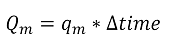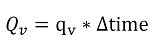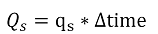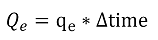# Generic Flowrate Totalisation

## Mass Total

For the number of Mass Totals selected by the user the following calculation is carried out:Symbol Description Units
Qm Mass total kg
qm Mass flowrate kg/s
Δtime Elapsed time s

## Gross Volume Total

For the number of Gross Volume Totals selected by the user the following calculation is carried out:Symbol Description Units
Qv Gross volume total m3
qm Gross volume flowrate m3/s
Δtime Elapsed time s

## Standard Volume Total

For the number of Standard Volume Totals selected by the user the following calculation is carried out:Symbol Description Units
Qs Standard volume total m3
qs Standard volume flowrate m3/s
Δtime Elapsed time s

## Energy Total

For the number of Energy Totals selected by the user the following calculation is carried out:Symbol Description Units
Qe Energy total GJ
qe Energy flowrate GJ/s
Δtime Elapsed time s